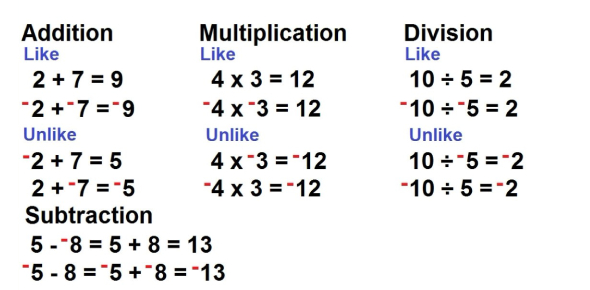# Integer Operations Trivia Test: Quiz

Approved & Edited by ProProfs Editorial Team
At ProProfs Quizzes, our dedicated in-house team of experts takes pride in their work. With a sharp eye for detail, they meticulously review each quiz. This ensures that every quiz, taken by over 100 million users, meets our standards of accuracy, clarity, and engagement.
| Written by Mrsrowland
M
Mrsrowland
Community Contributor
Quizzes Created: 3 | Total Attempts: 1,941
Questions: 10 | Attempts: 1,671Settings.

• 1.

### 7 * (-3)=

Explanation
When multiplying a positive number by a negative number, the result is always negative. In this case, when 7 is multiplied by -3, the answer is -21.

Rate this question:

• 2.

### 5 + (-3)=

Explanation
The given question is asking for the sum of 5 and -3. When we add a positive number and a negative number, we can think of it as subtracting the absolute value of the negative number from the positive number. In this case, we are subtracting 3 from 5, which gives us 2. Therefore, the correct answer is 2.

Rate this question:

• 3.

### -4 - 9=

Explanation
The given equation is -4 - 9. To solve this, we subtract 9 from -4. Subtracting a positive number from a negative number results in a more negative number. So, -4 - 9 equals -13.

Rate this question:

• 4.

### 20 / -2=

Explanation
The given expression represents the division of 20 by -2. When we divide a positive number by a negative number, the result is always negative. Therefore, the answer to 20 divided by -2 is -10.

Rate this question:

• 5.

### (-7)(-8)=

Explanation
When multiplying two negative numbers, the result is always positive. In this case, multiplying -7 by -8 gives us a positive result of 56.

Rate this question:

• 6.

### -12 / -4=

Explanation
The given expression is -12 divided by -4. When dividing two negative numbers, the result is always positive. Therefore, -12 divided by -4 equals 3.

Rate this question:

• 7.

### -8 + (-7)=

Explanation
The given expression involves adding two negative numbers, -8 and -7. When adding negative numbers, the result is always negative. In this case, -8 plus -7 equals -15.

Rate this question:

• 8.

### -4 - (-4)=

Explanation
When subtracting a negative number from another negative number, it is equivalent to adding the absolute values of the two numbers. In this case, the absolute value of -4 is 4. So, -4 - (-4) becomes -4 + 4, which equals 0.

Rate this question:

• 9.

### (-13) + 20=

Explanation
The given equation (-13) + 20 equals 7. To solve this equation, we start by adding -13 and 20. When we add these two numbers, we get 7 as the result. Therefore, the correct answer is 7.

Rate this question:

• 10.

### 3 - (-9)=

Explanation
The given equation is a subtraction problem, where we are subtracting a negative number (-9) from a positive number (3). When we subtract a negative number, it is equivalent to adding its absolute value. So, subtracting -9 is the same as adding 9. Therefore, 3 - (-9) is equal to 3 + 9, which equals 12.

Rate this question:

Related TopicsBack to top
×

Wait!
Here's an interesting quiz for you.